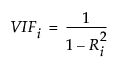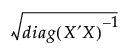Publication date: 05/24/2021

## Parameter Estimates

The Parameter Estimates report shows the estimates of the model parameters and, for each parameter, gives a t test for the hypothesis that it equals zero.

Note: Estimates are obtained and tested, if possible, even when there are linear dependencies among the model terms. Such estimates are labeled Biased or Zeroed. See Models with Linear Dependencies among Model Terms.

Term

The model term corresponding to the estimated parameter. The first term is always the intercept, unless the No Intercept option was checked in the Fit Model launch window. Continuous effects appear with the name of the data table column. Note that continuous columns that are part of higher order terms might be centered. Nominal or ordinal effects appear with values of levels in brackets. See Coding for Nominal Effects and The Factor Models for information about the coding of nominal and ordinal terms.

Estimate

The parameter estimates for each term. These are the estimates of the model coefficients. When there are linear dependencies among model terms, these might be labeled as Biased or Zeroed. See Models with Linear Dependencies among Model Terms.

Std Error

The estimates of the standard errors for each of the estimated parameters.

t Ratio

The tests of whether the true value of each parameter is zero. The t Ratio is the ratio of the estimate to its standard error. Given the usual assumptions about the model, the t Ratio has a Student’s t distribution under the null hypothesis.

Prob>|t|

The p-value for the test that the true parameter value is zero, against the two-sided alternative that it is not.

Lower 95%

The lower 95% confidence limit for the parameter estimate. This column appears only if you have the Regression Reports > Show All Confidence Intervals option selected or if you right-click in the report and select Columns > Lower 95%.

Upper 95%

The upper 95% confidence limit for the parameter estimate. This column appears only if you have the Regression Reports > Show All Confidence Intervals option selected or if you right-click in the report and select Columns > Upper 95%.

Std Beta

The parameter estimates for a regression model where all of the terms have been standardized to a mean of 0 and a variance of 1. This column appears only if you right-click in the report and select Columns > Std Beta.

VIF

The variance inflation factor for each term in the model. High VIFs indicate a collinearity issue among the terms in the model.

The VIF for the ith term, xi, is defined as follows:where Ri 2 is the RSquare, or coefficient of multiple determination, for the regression of xi as a function of the other explanatory variables. This column appears only if you right-click in the report and select Columns > VIF.

Design Std Error

The square roots of the relative variances of the parameter estimates (Goos and Jones 2011, p. 25):These are the standard errors divided by RMSE. This column appears only if you right-click in the report and select Columns > Design Std Error.